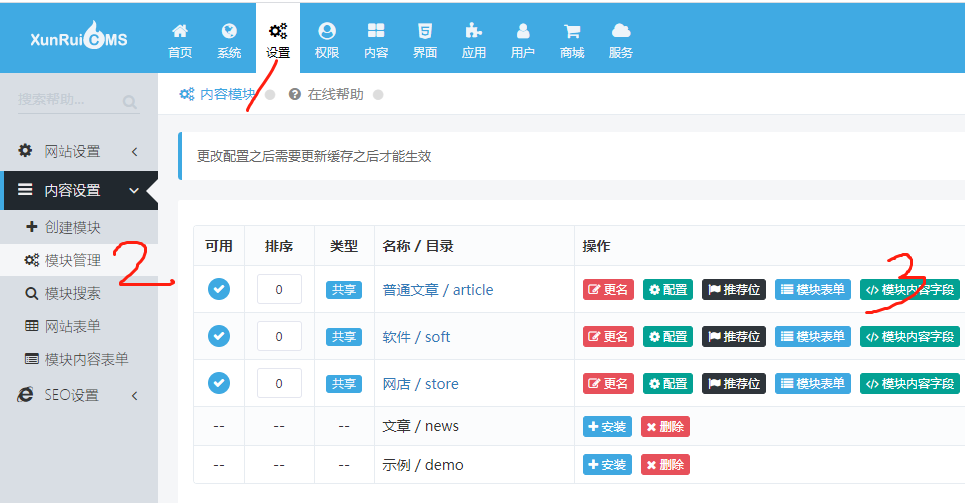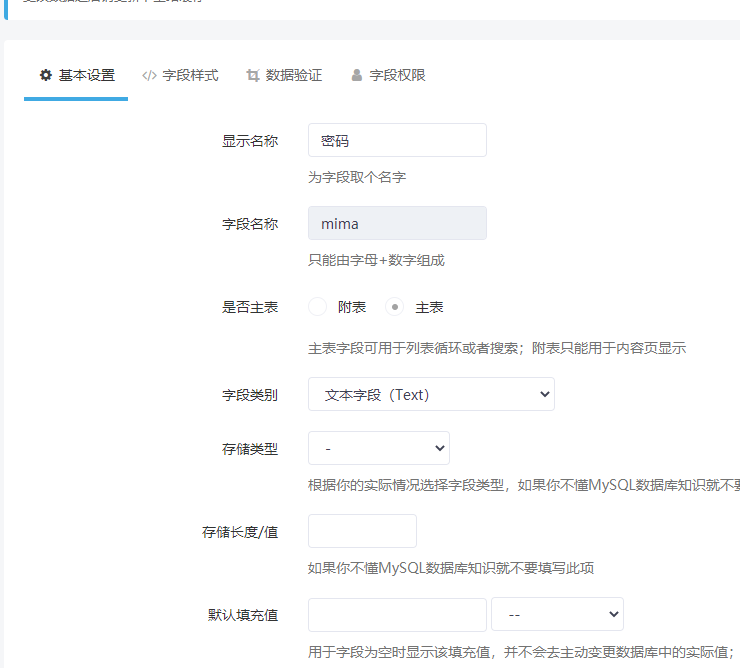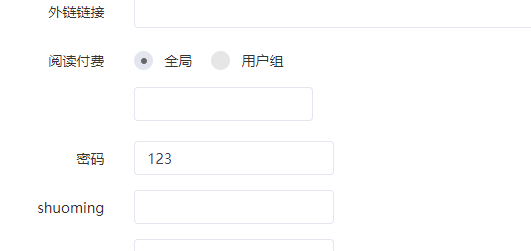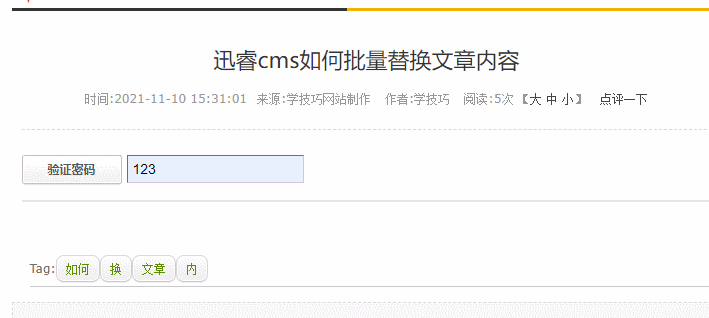# 迅睿CMS：xunruicms如何给文章设置密码访问？\dayrui\App\News\Controllers\Mima.php

```<?php namespace Phpcmf\Controllers;

/**
* 二次开发时可以修改本文件，不影响升级覆盖
*/

// 小波工作室二次开发
class Mima extends \Phpcmf\Common
{

public function index() {

\$id = (int)\$_GET['id'];
\$pwd = trim(\$_GET['pwd']);
\$this->_module_init();
\$data = \$this->content_model->get_data(\$id);
if (!\$data) {
\$this->_json(0, dr_lang('%s内容(#%s)不存在', \$this->module['name'], \$id));
}
if (\$pwd!=\$data['mima']) {
\$this->_json(0, '密码不正确');
}

\$this->_json(1, html_entity_decode(\$data['content']));

}

}```

Mima.php如果没有就自行创建一个。

```	<p>
{if \$mima}
<div class="input-group" id="mima_form">
<input type="text" id="xb_mima" value="" class="text1">
<span class="input-group-btn"><a class="button1" href="javascript:xb_mima();">验证密码</a></span>
</div>
<div id="neirong"></div>
<script>
function xb_mima() {
\$.get("{dr_url_prefix('index.php')}?is_ajax=1&s={MOD_DIR}&c=mima&id={\$id}&pwd="+\$("#xb_mima").val(), function(data){
if (data.code) {
\$('#neirong').html(data.msg);
\$("#mima_form").hide();
} else {
dr_cmf_tips(0, data.msg);
}
}, 'json');
}
</script>
{else}
{\$content}
{/if}
</p>```PS:

Tag:文章访问密码debug诊断信息需要在index.php文件中开启开发者模式才能查看
• 用微信  “扫一扫”

将文章分享到朋友圈。

关注公众号:xue-jiqiao